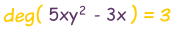# Degree (of an Expression)

"Degree" can mean several things in mathematics:

• In Geometry a degree (°) is a way of measuring angles,
• But here we look at what degree means in Algebra.

In Algebra "Degree" is sometimes called "Order"

## Degree of a Polynomial (with one variable)

A polynomial looks like this:example of a polynomial this one has 3 terms

The Degree (for a polynomial with one variable, like x) is:

the largest exponent of that variable.### More Examples:

 4x The Degree is 1 (a variable without an exponent actually has an exponent of 1) 4x3 − x + 3 The Degree is 3 (largest exponent of x) x2 + 2x5 − x The Degree is 5 (largest exponent of x) z2 − z + 3 The Degree is 2 (largest exponent of z)

## Names of Degrees

When we know the degree we can also give it a name!

Degree Name Example
0 Constant 7
1 Linear x+3
3 Cubic x3−x2+5
4 Quartic 6x4−x3+x−2
5 Quintic x5−3x3+x2+8

Example: y = 2x + 7 has a degree of 1, so it is a linear equation

Example: 5w2 − 3 has a degree of 2, so it is quadratic

Higher order equations are usually harder to solve:

• Linear equations are easy to solve
• Quadratic equations are a little harder to solve
• Cubic equations are harder again, but there are formulas to help
• Quartic equations can also be solved, but the formulas are very complicated
• Quintic equations have no formulas, and can sometimes be unsolvable!

## Degree of a Polynomial with More Than One Variable

When a polynomial has more than one variable, we need to look at each term. Terms are separated by + or - signs:example of a polynomial with more than one variable

For each term:

• Find the degree by adding the exponents of each variable in it,

The largest such degree is the degree of the polynomial.

### Example: what is the degree of this polynomial:Checking each term:

• 5xy2 has a degree of 3 (x has an exponent of 1, y has 2, and 1+2=3)
• 3x has a degree of 1 (x has an exponent of 1)
• 5y3 has a degree of 3 (y has an exponent of 3)
• 3 has a degree of 0 (no variable)

The largest degree of those is 3 (in fact two terms have a degree of 3), so the polynomial has a degree of 3

### Example: what is the degree of this polynomial:

4z3 + 5y2z2 + 2yz

Checking each term:

• 4z3 has a degree of 3 (z has an exponent of 3)
• 5y2z2 has a degree of 4 (y has an exponent of 2, z has 2, and 2+2=4)
• 2yz has a degree of 2 (y has an exponent of 1, z has 1, and 1+1=2)

The largest degree of those is 4, so the polynomial has a degree of 4

## Writing it Down

Instead of saying "the degree of (whatever) is 3" we write it like this:## When Expression is a Fraction

We can work out the degree of a rational expression (one that is in the form of a fraction) by taking the degree of the top (numerator) and subtracting the degree of the bottom (denominator).

Here are three examples:

## Calculating Other Types of Expressions

We can sometimes work out the degree of an expression by dividing ...

• the logarithm of the function by
• the logarithm of the variable

... then do that for larger and larger values, to see where the answer is "heading".

(More correctly we should work out the Limit to Infinity of ln(f(x))/ln(x), but I just want to keep this simple here).

 Note: "ln" is the natural logarithm function.Here is an example:

### Example: What is the degree of(3 plus the square root of x) ?

Let us try increasing values of x:

x ln() ln(x) ln()
/ ln(x)
2 1.48483 0.69315 2.1422
4 1.60944 1.38629 1.1610
10 1.81845 2.30259 0.7897
100 2.56495 4.60517 0.5570
1,000 3.54451 6.90776 0.5131
10,000 4.63473 9.21034 0.5032
100,000 5.76590 11.51293 0.5008
1,000,000 6.91075 13.81551 0.5002

Looking at the table:

• as x gets larger then ln() / ln(x) gets closer and closer to 0.5

So the Degree is 0.5 (in other words 1/2)

(Note: this agrees nicely with x½ = square root of x, see Fractional Exponents)

## Some Degree Values

Expression Degree
log(x) 0
ex
1/x −11/2